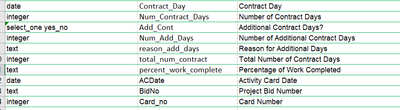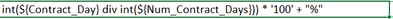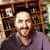# Percentage Calculation giving error

249
2
09-07-2021 07:45 PMNew Contributor III

I am currently the hunt for a solution to a very frustrating problem. I have a field that needs to simply divide two fields and return a percentage. Below are the screenshots of my calculations and fields. I need to divide Contract_Day by Total_Num_Contract and make sure to return a percentage sign. Third screenshot is the output.Tags (1)
2 RepliesbyMVP Esteemed Contributor

Contract_Day is a date? dividing a date isn't going to go well in any language expression

... sort of retired...byEsri Notable Contributor

As @DanPatterson points out you can not divide a date field by an integer and get the value you are wanting of percentage of days. Did you mean you want to divide by Num_Contract_Days by total_num_contract and convert to percent? That should be possible as both are integer values.

Can you share your XLSForm (xlsx file and any media folder files) so we can take a look at how you have configured the rest your calculations and the data types?

Also note your current calculation will not work, you are trying to do maths expressions and concatenate strings in the same calculation. You need to split this out into two seperate calculations (you can make them hidden). So that you calculate your final value, just a integer or decimal. And then in next calculation you can concatenate the integer and string together to get the text represenation you are after.

Regards,

Phil.